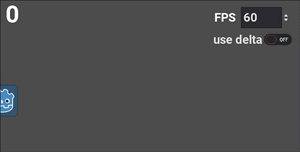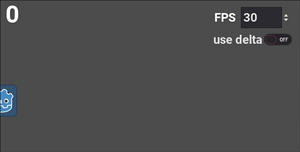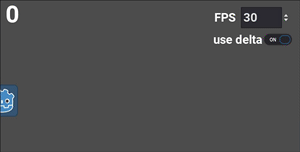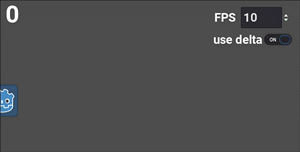# Understanding 'delta'

## Problem

The `delta` or “delta time” parameter is a frequently-misunderstood concept in game development. In this tutorial, we’ll explain how it’s used, the importance of frame-rate independent movement, and practical examples of its use in Godot.

## Solution

To illustrate the problem, let’s consider a `Sprite` node moving across the screen. If our screen is `600` pixels wide and we want the sprite to take `5` seconds to cross the screen, we can use the following calculation to find the necessary speed:

``````600 pixels / 5 seconds = 120 pixels/second
``````

We’ll move the sprite every frame using the `_process()` function. If the game is running at `60` frames per second, we can find the per-frame movement like so:

``````120 pixels/second * 1/60 second/frame = 2 pixels/frame
``````

Here’s the necessary code:

``````extends Node2D

# Desired movement in pixels/frame
var movement = Vector2(2, 0)

func _process(delta):
\$Sprite.position += movement
``````

Run this code and you’ll see the sprite takes 5 seconds to cross the screen.Maybe. The trouble begins if there is something else occupying the computer’s time. This is called lag and can come from a variety of sources - the cause could be your code or even other applications running on your computer. If this happens, then the length of a frame might increase. As an extreme example, imagine that the frame rate is halved - each frame took 1/30 instead of 1/60 of a second. Moving at `2` px/frame, it’s now going to take twice as long for the sprite to reach the edge.Even small frame rate fluctuations will result in inconsistent movement speed. If this were a bullet or other fast-moving object, we wouldn’t want it slowing down like this. We need the movement to be frame rate independent.

### Fixing the frame rate problem

When using the `_process()` function, it automatically includes a parameter called `delta` that’s passed in from the engine (so does `_physics_process()`, which is used for physics-related code). This is a floating point value representing the length of time since the previous frame. Typically, this will be approximately 1/60 or `0.0167` seconds.

With this information, we can stop thinking about how much to move each frame, and only consider our desired speed in pixels/second (`120` from the above calculation).

Multiplying the engine’s `delta` value by this number will give us how many pixels to move each frame. The number will automatically adjust if the frame time fluctuates.

``````# 60 frames/second
120 pixels/second * 1/60 second/frame = 2 pixels/frame

# 30 frames/second
120 pixels/second * 1/30 second/frame = 4 pixels/frame
``````

Note that if the frame rate decreases by half (meaning the frame time doubles), then our per-frame movement must also double to keep the desired speed.

Let’s change the code to use this calculation:

``````extends Node2D

# Desired movement in pixels/second.
var movement = Vector2(120, 0)

func _process(delta):
\$Sprite.position += movement * delta
``````

Now when running at `30` frames per second, the travel time is consistent:If the frame rate gets very low, the movement is no longer smooth, but the time remains the same.### Using delta with motion equations

What if your movement is more complex? The concept remains the same. Keep your units in seconds, not frames, and multiply by `delta` each frame.

For example, if you are applying a gravity, that’s an acceleration - each frame it will increase the velocity by some amount. As in the above example, the velocity then changes the node’s position.

Try adjusting `delta` and `target_fps` in the following code to see the effect:

``````extends Node2D

# Acceleration in pixels/sec/sec.
var gravity = Vector2(0, 120)
# Acceleration in pixels/frame/frame.
var gravity_frame = Vector2(0, .033)

# Velocity in pixels/sec or pixels/frame.
var velocity = Vector2.ZERO

var use_delta = false
var target_fps = 60

Engine.target_fps = target_fps

func _process(delta):
if use_delta:
velocity += gravity * delta
\$Sprite.position += velocity * delta
else:
velocity += gravity_frame
\$Sprite.position += velocity
``````

Note that we’re multiplying by our timestep each frame to update both `velocity` and `position`. Any quantity that is updated every frame should be multiplied by `delta` to ensure it changes independent or frame rate.

#### Using kinematic functions

In the above examples, we’ve used a `Sprite` to keep things simple, updating the `position` every frame. If you’re using a kinematic body (in 2D or 3D), you’ll instead be using one of its movement methods. Specifically in the case of `move_and_slide()`, there tends to be some confusion, because it automatically applies `delta` to the movement vector. This means you won’t multiply your velocity by `delta` . But you will still need to apply it on the acceleration. For example:

``````# Sprite movement code:
velocity += gravity * delta
position += velocity * delta

# Kinematic body movement code:
velocity += gravity * delta
velocity = move_and_slide(velocity)
``````

If you don’t use `delta` when applying acceleration to your velocity, then your acceleration will be subject to fluctuations in frame rate. This can have a_much more subtle effect on movement - it will be inconsistent, but much more difficult to diagnose.